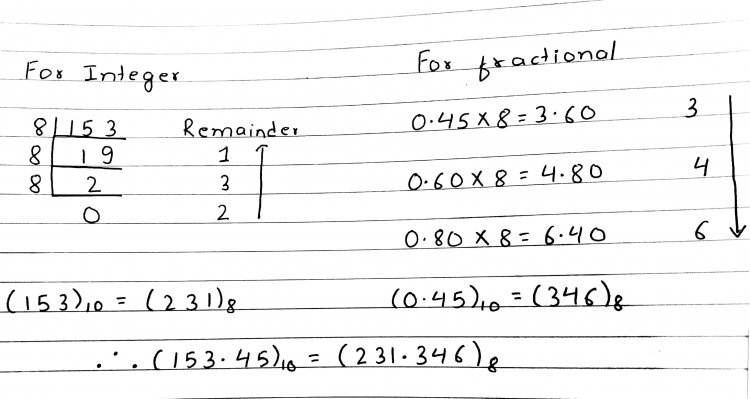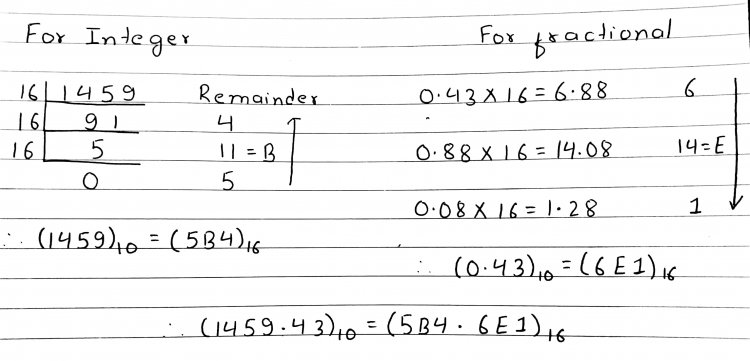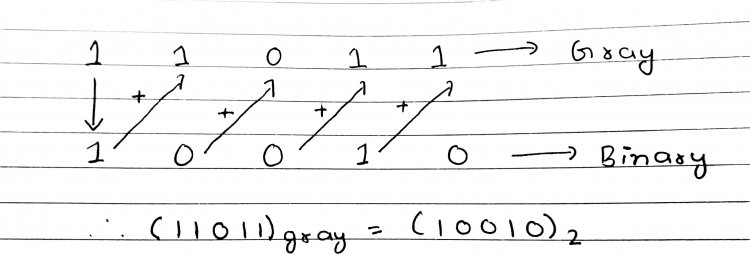# Number System2.1  Binary, Octal & Hexadecimal Number Systems and Their Conversion

Number systems are the technique to represent numbers in the computer system architecture.

Computer architecture supports following number systems.

1. Binary number system
2. Decimal number system
3. Octal number system

Base/Radix(r): It is number of digits used to represent particular number system.

For radix  r, we have values from 0 to (r-1) to represent number system.

• A Binary number system has only two digits that are 0 and 1(0 to 2-1).
• Every number (value) represents with 0 and 1 in this number system.
• The base of binary number system is 2, because it has only two digits.

• Decimal number system has only ten (10) digits from0 to 9.
• Every number (value) represents with 0,1,2,3,4,5,6, 7,8 and 9 in this number system.
• The base of decimal number system is 10, because it has only 10 digits.

• Octal number system has only eight (8) digits from0 to 7.
• Every number (value) represents with 0,1,2,3,4,5,6 and 7 in this number system.
• The base of octal number system is 8, because it has only 8 digits.

• A Hexadecimal number system has sixteen (16) alphanumeric values from 0 to 9and A to F.
• Every number (value) represents with 0,1,2,3,4,5,6, 7,8,9,A,B,C,D,E and F in this number system.
• The base of hexadecimal number system is 16, because it has 16 alphanumeric values. Here A is 10B is 11C is 12D is 13E is 14and F is 15.

2.1 Conversion from one number system to others

Conversion from binary to decimal:

(10010110)2
= 1x27+0x26+0x25+1x24+0x23+1x22+1x21+0x20
= 128+0+0+16+0+4+2+0
= (150)10

(1010.01)2
= 1×23 + 0x22 + 1×21+ 0x20 + 0x2 -1 + 1×2 -2
= 8+0+2+0+0+0.25
= (10.25)10

Conversion from binary to octal:

(1010111100)2
= (001 010 111 100)2
= (1 2 7 4)8
= (1274)8

(0110 011.1011)2
= (0 110 011 . 101  1)2
= (110  011 . 101  100)2
= (6  3 . 5  4)8
= (63.54)8

(1010101101001)2
= (1  0101  0110  1001)2
= (0001  0101  0110  1001)2
= (1  5  6  9)16
= (1569)16

(001100101.110111)2
= (0  0110  0101 . 1101  1100)2
= (0110  0101 . 1101  1100)2
= (6  5 . D  C)16
= (65.DC)16

Conversion of decimal to binary:

(41.6875)10 = (?)2

Here, Integer part = 41  and fractional part = 0.6875(25.625)10  = (11001.101)2

Conversion of decimal to octal:

(153.45)10 = (?)8

Here, integer part=153 and fractional part is 0.45Conversion of decimal to hexa-decaimal:

(1459.43)10 = (?)16

Here, integer part =1459 and fractional part =0.43Conversion from octal to binary:

• Take each digit individually as decimal digit and convert into binary and place it in the combination of 3 bit each.

(540)8
= (101 100 000)2
= (101100000)2

(352.563)8
= (011 101 010 . 101 110 011)2
= (011101010.101110011)2

(673.12)8
= 110  111  011. 001  010
= (110111011.00101)2

Conversion from octal to decimal:

• Write the octal digit as power of 8 increasing from right to left starting from 0. if fraction arise write as negative power of 8 from left to right starting from point as -1,-2 and so on.
• Convert each power of 8 into its decimal form.
• Add these terms to give the decimal number.

(125)8

= 1*82+ 2*81 + 5*80
= 64+16+5
= (25)10

(0.14)8
= 1*8-1+ 4*8-2
= (0.1875)10

(127.54)8
= 1*82+ 2*81 + 7*80+ 5*8-1 + 4*8-2
=64+16+7+0.625+0.0625
=(87.6875)10

• This cannot be changed directly.
• First we need to convert the given octal into decimal/binary and then to hexadecimal.

(56)8
= (101)(110)
= (101110)2
= (10)(1110)
= (2)(14)
= (2E)16

(1745.246)8

Ans. (3E5.530)16

• first change to binary system and then to hexadecimal

Conversion from Hex to binary:

• Convert each digit individually to its binary equivalent and place in combination of 4 bits.

(3A6.C)16
= 0011 1010 0110. 1100
= (1110100110.11)2

Conversion from Hex to decimal:

(B65F)16
= B*163+6*162+5*161+F*16o
= 11*163+6*162+5*161+15*16o
=(46687)10

Conversion from hex to octal:

• Convert hex to binary/decimal and then to octal

(AF.2B)16
= 1010  1111 . 0010 1011
=  010 101 111. 001 010 110
= (257.126)8

(3E5.530)16

Ans.       (1745.246)8

• First change to binary system and then to octal system.

2.2 Representation of BCD, ASCII, Excess-3, Gray Code, Error Detection and Correcting Codes.

In the digital coding, numbers or letters are represented by a specific group of symbols, such group of symbols are called as “Code”.

The digital data is represented, stored and transmitted as group of bits. This group of bits is also called as Digital Codes(binary codes).

• suitable for the computer applications.
• suitable for the digital communications.
• make the analysis and designing of digital circuits.
• only 0 & 1 are being used, implementation becomes easy.

Classification of Digital Codes:

• Weighted Codes ( binary, BCD)
• Non-Weighted Codes (Excess-3, Gray)
• Alphanumeric Codes (ASCII code)
• Error Detecting Codes(parity code)
• Error Correcting Codes(Hamming code)

Weighted Codes:

• Weighted codes are those which obey the positional weight principle. Each position of the number represents a specific weight.
• e.g. BCD(8421) code ,2421 code etc.

1.Binary Coded Decimal (BCD) code

• In this code each decimal digit is represented by a 4-bit binary number. So, with four bits we can represent sixteen numbers from (0000 to 1111).
• But in BCD code only first ten of these are used (0000 to 1001) which represents one of the ten decimal digits from 0 to 9.
• The remaining six code combinations i.e. 1010 to 1111 are invalid in BCD.

e.g. (185)10 = (0001 1000 0101)BCD = (10111001)2

• It is very similar to decimal system.
• It is simple to implement a hardware algorithm for BCD converter.

• The addition and subtraction of BCD have different rules.
• BCD needs more number of bits than binary to represent the decimal number. So, BCD is less efficient than binary number system. E.g. to represent 13 in binary – 1101 but in                 BCD code – 0001 0011

Non-Weighted Codes:

• In such code the positional weights are not assigned.
• Here, digit value does not depend upon their position i.e., each digit position within the number is not assigned fixed value.
• Example of non-weighted codes are: Excess-3 code and gray code.

Excess-3 code:

• It is a type of non-weighted code .
• Also called as XS-3 code.
• The Excess-3 code words are derived from the 8421 BCD code words adding (0011)2 or (3)10.
• It is only the un-weighted code that is self-complementing.

Example1:

Convert  12 to an excess-3 number.

First, add 3 to each decimal digit i.e. 1+3=4 and 2+3=5

Second, convert sum to BCD form i.e. 0100  0101

So, 01000101 is excess-3 code for decimal 12.

Example2:

Convert  29 to an excess-3 number.

First,  2+3=5 and 9+3=12

 2 9 +3 +3 =5 =12

After adding 9 and 3, do not carry the 1 into the next column; instead leave the result intact as 12, and then convert.

Second, 0101 and 1100

So, 01011100 is excess-3 code for decimal 29.

Gray Code:

• It is the non-weighted code
• Only one bit will change each time the decimal number is incremented thus, the gray code is called a unit distance code.
• Gray code is popularly used in the shaft position encoders. A shaft position encoder produces a code word which represents the angular position of the shaft.

Binary to Gray Code conversion:

1. Keep MSB of gray code same as binary code.
2. Going from left to right add adjacent pairs of binary code to get gray code bit.

E.g. Convert 10110 to gray code.

 1+ 0+ 1+ 1+ 0 ↓ ↓ ↓ ↓ ↓ 1 1 1 0 1

E.g. Convert (1010011)2 in gray code. (Ans. 1111010)

E.g. What is gray code for decimal number 15? (Ans. 1000)

Gray to binary conversion:

1. Keep MSB of binary same as of gray code.
2. Add each binary code generated to gray code bit in the next adjacent.

E.g. Convert 11011 gray code to binary.ASCII Code:

• Stands for Abbreviation for American Standard Code for Information Exchange.
• ASCII is universally accepted alpha-numerical code used in most computers and other electronic equipment.
• Most computer keyboards are standardized with the ASCII code.
• It is a 7-bit code. So, it represents 128 characters.
• e.g. ‘a’=9710=1100001

‘A’=6510=1000001

Error Detection Codes

During the process of binary data transmission error may occur. Noise can cause error in binary bits i.e. if ‘0’ is changed to ‘1’ or ‘1’ is changed to ‘0’. So this error must be detected.

Error detection is the process of detecting the errors that are present in the data transmitted from transmitter to receiver, in a communication system.

We use some redundancy codes to detect these errors, by adding to the data while it is transmitted from source . It is done by use of error detecting codes.

Types of Error Detection Codes are:

1. Parity Check
2. Cyclic Redundancy Check (CRC)
3. Check Sum

Parity Check:

A parity bit is an extra bit included with binary message to make the number of 1’s either even or odd.

A parity bit is used to detect error during transmission of binary information.

Even parity: No of 1’s in given word including parity should be even.

Odd parity: No of 1’s in given word including parity should be odd.

E.g.  Message is 101

For odd parity  1011

For even parity 1010

Also, if given data to be sent is 1001011. we send this data after adding parity (let us assume we made it as even parity 01001011)

At receiver if bit changes (00001011) error is seen.

Limitations of parity checking:

1. The bit in which the error occur cannot be detected.
2. Not suitable for detecting multiple errors.(00101011)
3. This method cannot correct the error.

CRC

This is based on concept of binary division.

CRC generator:

First we need to generate CRC. Let us assume we have a data unit.

1. Append the string of ‘n’ 0’s to data unit.
2. Divide newly generated data unit by the divisor. It must be ‘n+1’ bits.
3. The remainder we obtain is ‘n’ bit CRC.
4. The ‘n’ number of 0’s are replaced by CRC to get code word to be transmitted to the receiver side.

CRC checker:

At receiver side there will be CRC checker.

1. It divides the data unit by the same divisor which was used by the transmitter.
2. The remainder of the result is checked. If remainder is ‘0’, there is no error (accepted). If remainder is ‘not 0’, there is some error(rejected)

Data unit = 100100  divisor= 1101

Check Sum:

Here, each word is added to previous word and total sum(checksum) is calculated. Thus, the checksum is transmitted along with the data.

E.g. (7,11,12,0,6) data is to be sent.

Here, checksum is 7+11+12+0+6 = 36

At Transmitter, data along with checksum is send

At Receiver, again checksum is calculated to ensure the same data which was transmitted is received.

Error correcting codes:

• They are used to correct the errors present in the received data bit stream so that, we get the original data.
• E.g. Hamming code.

4.4  Addition and Subtraction of Binary Numbers

Binary addition is key to binary subtraction, multiplication and division.

The basic rule for binary addition are:

 0+0=0 Sum=0 Carry=0 0+1=1 Sum=1 Carry=0 1+0=1 Sum=1 Carry=0 1+1=10 Sum=0 Carry=1 1+1+1=11 Sum=1 Carry=1

Binary Subtraction:

Rule for binary subtraction are:

 1-1=0 Difference=0 Borrow=0 1-0=1 Difference=1 Borrow=0 0-1=1 Difference=1 Borrow=1 0-0=0 Difference=0 Borrow=0

Complements

Complements are used in digital computers to simplify the subtraction operation and for logical manipulation.

There are two types of complements for each base r system:

The r’s Complement

r’s complement of N = rn – N
where, n = no. of digits, N = Number, r = radix

Examples:

1. Find 10’s complement of (52520)10
Ans. 10’s complement of (52520)10 = 105 - 52520= 47480
1. Find 10’s complement of (305)10
Ans. 10’s complement of (305)10 = 103 - 305 = 1000-305= 695
1. Find 10’s complement of (0.252)10
Ans. As there is no integer part, n=0
10’s complement of (0.252)10 = 10n - N= 100– 0.252= 1-0.252=0.748
1. Find 2’s complement of (10101)2
Ans. 2’s complement of (10101)2 = 25 – 10101 = 100000-10101= 1011
1. Find 2’s complement of (0.0110)2
Ans. 2’s complement of (0.0110)2 = 20 – 0.0110 = 1-0.0110= 0.1010

The (r-1)’s Complement

(r-1)’s complement of N = rn – r-m- N
where, n = no. of digits, N = Number, r = radix

1. Find 9’s complement of (52520)10
Ans. Here, n=5, m=0
9’s complement of (52520)10 = 105 – 100 - 52520 = 100000-1-52520 = 47479
2. Find 9’s complement of (0.252)10
Ans. Here, n=0, m=3
9’s complement of (0.252)10 = 100 – 10-3 - 0.252 = 1- 0.001-0.252 = 0.747
3. Find 9’s complement of (25.639)10
Ans. Here, n=2, m=3
9’s complement of (0.252)10 = 102 – 10-3 - 0.252 =100- 0.001-25.639 = 73.36
4. Find 1’s complement of (101100)2
Ans. Here, n=6, m=0

9’s complement of (101100)10

= 26 – 20  - 101100
=(26 –1)-101100
= 111111 – 101100
= 010011

5. Find 1’s complement of (0.0110)2

Ans. Here, n=0, m=4
9’s complement of (0.0110)10

= 20 – 2-4 – 0.0110
=(1 – 2-4  )– 0.0110
= 0.1111 – 0.0110
= 0.1001

Note: (1 – 2-4 ) = 0.9375  was converted into binary in the above.

2’s complement representation

In this representation, a negative number is represented 2's complement of its positive value.

To represent -14 with eight bits. In signed-2's complement representation 1 1110010

1’s complement: The 1’s complement of a binary number is the number that results when we complement each bit.

2’s complement: The 2’s complement is the binary number that results when we add 1 to the 1’s complement.
i.e. 2’s complement = 1’s complement + 1

Subtraction is done using 2’s complement method widely. This leads to the simplest logic circuits for performing arithmetic.

To represent -7 in signed 2’s complement .
+7 ⇒0000   0111
-7 ⇒ 1111   1000 +1  ⇒1111  1001